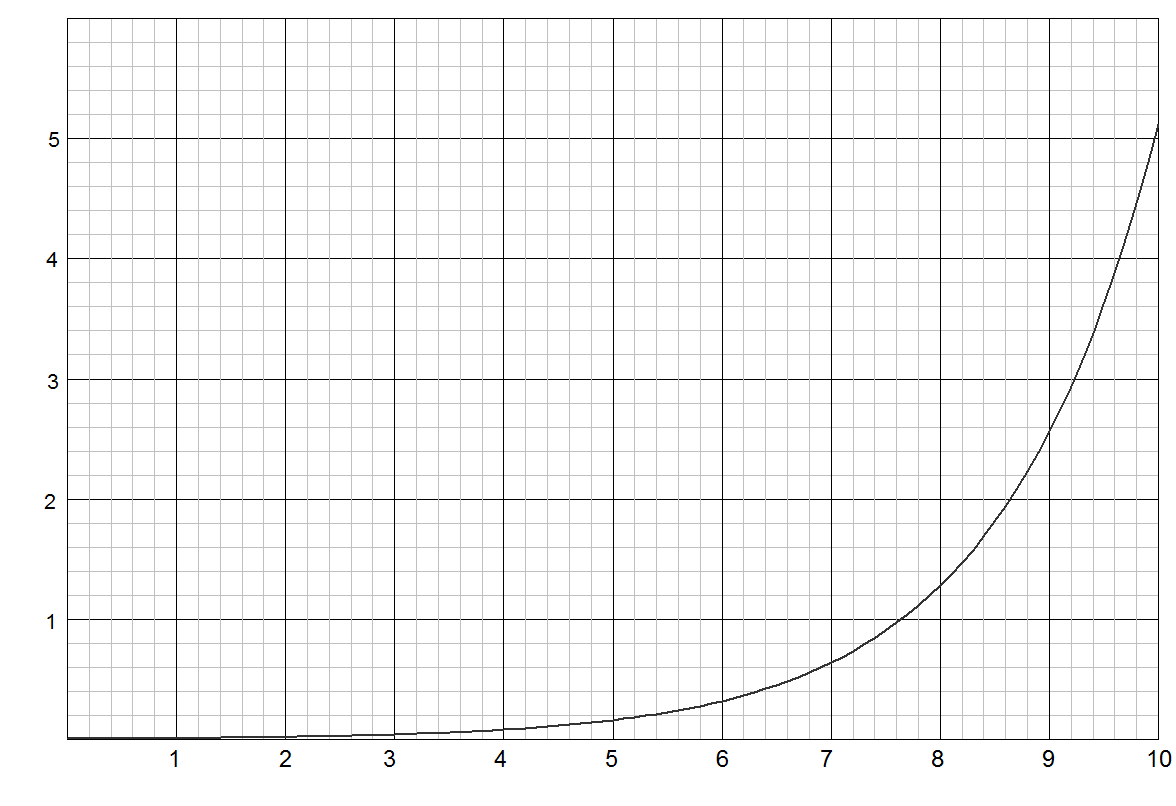# What Does That "Exp" Mean?

The "exp" stands for "exponential". The term "exp(x)" is the same as writing e^x or "e to the x" or "e to the power of x". In this context, "e" is a universal constant, e = 2.718281828... it goes on forever but you don't need to know the value, your calculator probably has exp(x) or e^x as a function (if, as I am assuming, it is a scientific calculator).

It might become obvious to you if you see the equations written properly rather than in ascii text. Check the quick reference page.

Where the single stage equations are also written by way of review. It might be easier for you to understand what is meant by exp(x) when you see it written properly as e^x.

The inverse of e^x is ln(x), or the natural logarithm of x. So in other words, if I take the natural logarithm of e^x, I get x back: in equation form ln(e^x) = x, or equivalently, ln(exp(x)) = x. It works the other way around, too, exp(ln(x)) = x.

The expression 1-exp(x) means raise the number e to the x power then subtract it from 1. So you would say it "one minus e to the x".

Relating to a mathematical expression containing one or more exponents. Something is said to increase or decrease exponentially if its rate of change must be expressed using exponents. A graph of such a rate would appear not as a straight line, but as a curve that continually becomes steeper or shallower.Information on this page can be used for educational purposes as long as this site is credited, the contents of this site can not be copied without our permission to other websites or for commercial gain.